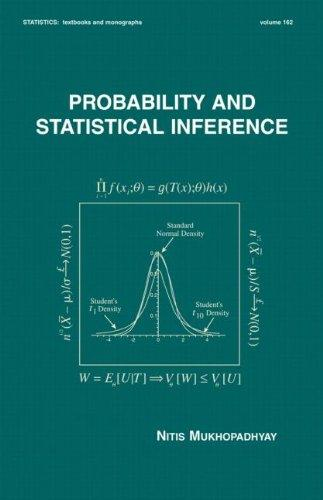188298

9780824703790

# Probability and Statistical Inference (Statistics: A Series of Textbooks and Monographs)Out of Stock

The item you're looking for is currently unavailable.

Most renters respond to questions in 48 hours or less.
The response will be emailed to you.
• ISBN-13: 9780824703790
• ISBN: 0824703790
• Edition: 1
• Publication Date: 2000
• Publisher: CRC Press

### SUMMARY

Priced very competitively compared with other textbooks at this level! This gracefully organized textbook reveals the rigorous theory of probability and statistical inference in the style of a tutorial, using worked examples, exercises, numerous figures and tables, and computer simulations to develop and illustrate concepts. Beginning with an introduction to the basic ideas and techniques in probability theory and progressing to more rigorous topics, Probability and Statistical Inference studies the Helmert transformation for normal distributions and the waiting time between failures for exponential distributions develops notions of convergence in probability and distribution spotlights the central limit theorem (CLT) for the sample variance introduces sampling distributions and the Cornish-Fisher expansions concentrates on the fundamentals of sufficiency, information, completeness, and ancillarity explains Basu's Theorem as well as location, scale, and location-scale families of distributions covers moment estimators, maximum likelihood estimators (MLE), Rao-Blackwellization, and the Cramèr-Rao inequality discusses uniformly minimum variance unbiased estimators (UMVUE) and Lehmann-Scheffè Theorems focuses on the Neyman-Pearson theory of most powerful (MP) and uniformly most powerful (UMP) tests of hypotheses, as well as confidence intervals includes the likelihood ratio (LR) tests for the mean, variance, and correlation coefficient summarizes Bayesian methods describes the monotone likelihood ratio (MLR) property handles variance stabilizing transformations provides a historical context for statistics and statistical discoveries showcases great statisticians through biographical notes Employing over 1400 equations to reinforce its subject matter, Probability and Statistical Inference is a groundbreaking text for first-year graduate and upper-level undergraduate courses in probability and statistical inference who have completed a calculus prerequisite, as well as a supplemental text for classes in Advanced Statistical Inference or Decision Theory.Nitis Mukhopadhyay is the author of 'Probability and Statistical Inference (Statistics: A Series of Textbooks and Monographs)', published 2000 under ISBN 9780824703790 and ISBN 0824703790.

You can find lots of answers to common customer questions in our FAQs

View a detailed breakdown of our shipping prices